# Square root + quadratic equation - math problems

#### Number of examples found: 35Solve quadratic equation: 2x2-58x+396=0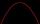Solve pure quadratic equation ?.
• Solve equationsolve equation: ?
• Factors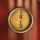Can the expression ? be factored into rational factors?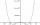By introducing a new variable solve biquadratic equation: ?
• Non linear eqsSolve the system of non-linear equations: 3x2-3x-y=-2 -6x2-x-y=-7
• Completing squareSolve the quadratic equation: m2=4m+20 using completing the square method
• ProductThe product of two consecutive odd numbers is 8463. What are this numbers?
• Three members GPThe sum of three numbers in GP (geometric progression) is 21 and the sum of their squares is 189. Find the numbers.
• Cuboid wallsIf the areas of three adjacent faces of a cuboid are 8 cm², 18 cm² and 25 cm². Find the volume of the cuboid.
• Unknown number 4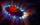I think number. When I divided its square root by its 1/9, I get a number 1. What number am I thinking?Find the radius of the circle with area S = 200 cm².
• Find parametersFind parameters of the circle in the plane - coordinates of center and radius: ?
• TrapeziumThe length of the base and the height size of the base of the trapezium is at ratio 5:3:2, the content area of the trapezium is 128 cm2. Calculate the length of the base and the height of the trapezoid.
• Rhombus and inscribed circleIt is given a rhombus with side a = 6 cm and the radius of the inscribed circle r = 2 cm. Calculate the length of its two diagonals.
• 1 page1 page is torn from the book. The sum of the page numbers of all the remaining pages is 15,000. What numbers did the pages have on the page that was torn from the book?
• Apples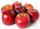A 2 kg of apples cost a certain sum of money. This sum is equal to the amount of kilograms for which we pay 72 CZK. How much is 1 kg of apples?
• 3d vector componentThe vector u = (3.9, u3) and the length of the vector u is 12. What is is u3?
• Unknown number 7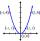Calculate unknown number whose 12th power when divided by the 9th power get a number 27 times greater than the unknown number. Determine the unknown number.
• TangentsTo circle with a radius of 41 cm from the point R guided two tangents. The distance of both points of contact is 16 cm. Calculate the distance from point R and circle centre.

Do you have an interesting mathematical word problem that you can't solve it? Submit a math problem, and we can try to solve it.

We will send a solution to your e-mail address. Solved examples are also published here. Please enter the e-mail correctly and check whether you don't have a full mailbox.

Please do not submit problems from current active competitions such as Mathematical Olympiad, correspondence seminars etc...
Looking for help with calculating roots of a quadratic equation?